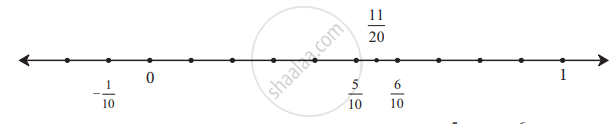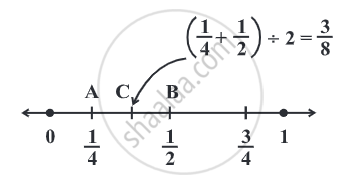# Rational Numbers between two rational numbers:

If m is an integer, then m + 1 is the next bigger integer. There is no integer between m and m + 1. The integers that lie between any two non-consecutive integers can be counted. However, there are infinitely many rational numbers between any two rational numbers.

There are unlimited numbers of rational numbers between two rational numbers.

List rational numbers between – 2 and – 1.

Let us write  – 1 and – 2 as rational numbers with denominator 5.

We have, - 1 = -5/5 and -2 = - 10/5

"So", -10/5 < -9/5 < -8/5 < -7/5 < - 6/5 < -5/5 or - 2 < -9/5 < -8/5 < -7/5 < - 6/5 < -1.

The rational numbers between –2 and –1 would be, (- 9)/5, (- 8)/5, (- 7)/5, (- 6)/5.

Taking another example, let us find numbers between the rational numbers 1/2 and 3/5.

Let us first convert each of the numbers into their equivalent fractions.

For example, 1/2 = 5/10, and 3/5 = 6/10On the number line, there are points showing the numbers 5/10 and 6/10.

Let us find the mid-point of the line segment joining these two points and see what number it shows.

1/2 (5/10 + 6/10) = 11/20

This is the mid-point of the line segment.

Because 6/10 - 11/20 = (12 - 11)/20 = 1/20 and

also 11/20 - 5/10 = (11 - 10)/20 = 1/20

Thus, 11/20  "is the number that lies exactly in the middle of" 5/10 and 6/10.

It means that, 11/20 "is a number that lies between"1/2 and 35.

Similarly, we can find numbers that lie between 1/2 and 11/20 "and between" 11/20 and 3/5.

#### Example

Write any 3 rational numbers between –2 and 0.

– 2 can be written as (-20)/10 "and"  0  "as"  0/10.

Thus we have (-19)/10, (-18)/10, (-17)/10, (-16)/10, (-15)/10, ..........., (-1)/10 between - 2 and 0.

#### Example

Find any ten rational numbers between (-5)/6 "and" 5/8.

We first convert (-5)/6 "and" 5/8 to rational numbers with the same denominators.
(-5 xx 4)/(6 xx 4) = (-20)/24  "and"  (5 xx 3)/(8 xx 3) = 15/24.

Thus we have (-19)/24, (-18)/24, (-17)/24, ........, (14)/24 as the rational numbers between(-20)/24  "and"  15/24.

#### Example

Find a rational number between 1/4 "and" 1/2.

(1/4 + 1/2) ÷ 2 = ((1 + 2)/4) ÷ 2 = 3/4 xx 1/2 = 3/8.

3/8 "lies between" 1/4 "and" 1/2.We find the midpoint of AB which is C, represented by
(1/4 + 1/2) ÷ 2 =  3/8.
1/4 < 3/8 < 1/2.
If you would like to contribute notes or other learning material, please submit them using the button below.

### Shaalaa.com

Finding Rational Numbers Between Two Rational Numbers- Theory [00:20:45]
S
0%